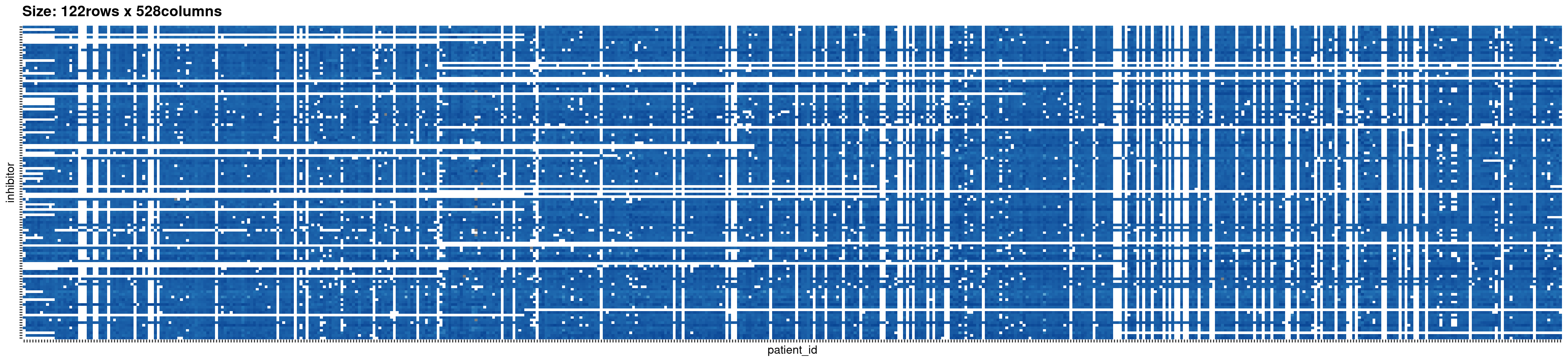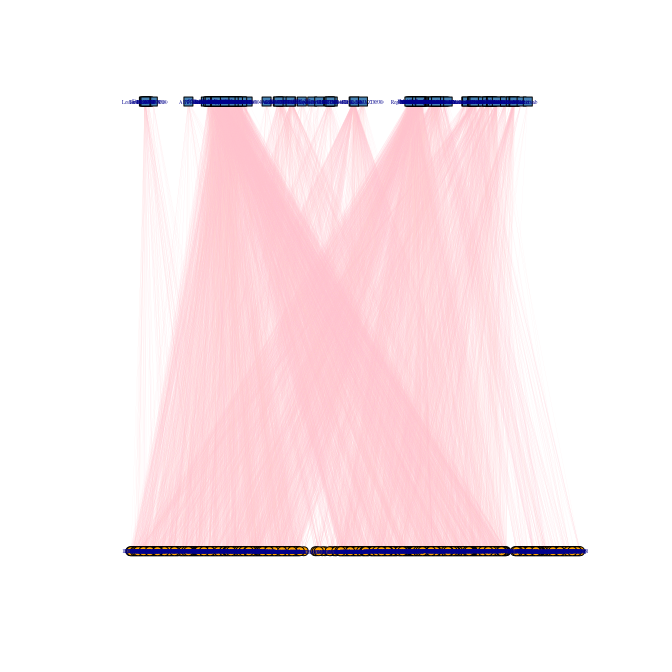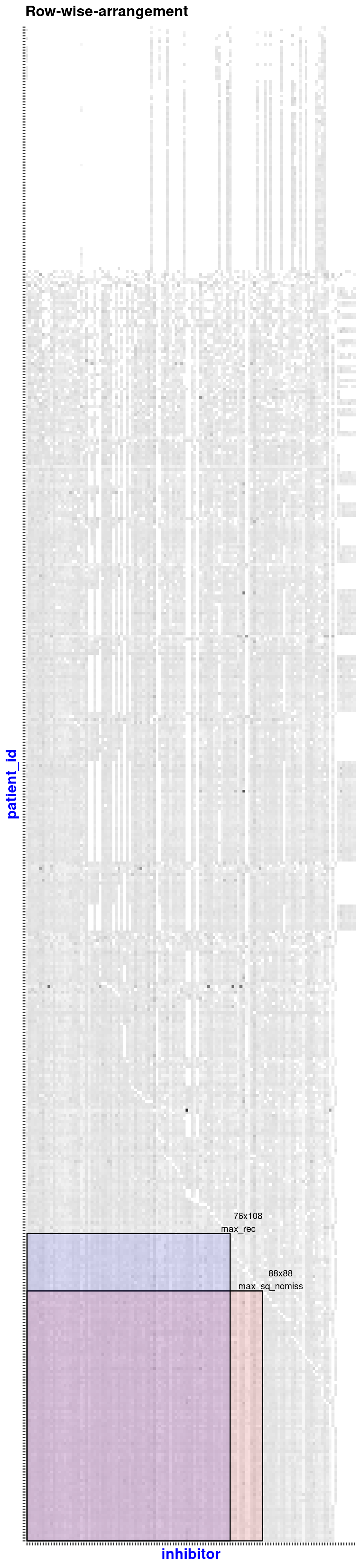NIMAA-vignette

Introduction

When dealing with nominal data, usually only some methods such as simple frequency statistics can be carried out. NIMAA package proposes a pipeline for nominal data mining, which can effectively find special relationships between data.

It uses bipartite graphs to represent the relationship between two different types of nominal data, and organizes them in the incidence matrix to find sub-matrices that are larger in different dimensions and do not contain any missing values.

Then, two one-partite graphs are obtained on the sub-matrix using a variety of different methods of projection. For any of them, NIMAA can use a variety of methods to cluster, and users can use possible external prior knowledge to select. the result of the best clustering method as the ‘reference cluster’.

After that we can perform multiple numerical imputations on the matrix with missing data, and apply the same clustering method as ‘reference clustering’ for clustering, and select the best data imputation method which is the one with the closest result to the ‘reference cluster’.

library(NIMAA)

1 Explore the data

Here we use ‘beatAML’ dataset as an example, which is a data set composed of three columns, the first two columns are nominal data, and the third column is numerical data.

beatAML dataset samples
inhibitor patient_id median
Alisertib (MLN8237) 11-00261 81.00097
Barasertib (AZD1152-HQPA) 11-00261 60.69244
Canertinib (CI-1033) 11-00261 87.03067
Crenolanib 11-00261 68.13586
CYT387 11-00261 69.66083
Dasatinib 11-00261 66.13318
Doramapimod (BIRB 796) 11-00261 101.52120
Dovitinib (CHIR-258) 11-00261 33.48040
Erlotinib 11-00261 56.11189

Read the data from the package:

beatAML_data <- NIMAA::beatAML

1.1 Plot the original data:

Function plotInput() will print the incidence matrix plot of input data, and return that matrix.

NB: To keep the size of vignette small enough for CRAN rules, we won’t output the interactive figure here.

beatAML_incidence_matrix <- plotInput(
x = beatAML_data, # original data with 3 columns
index_nominal = c(2,1), # the first two columns are nominal data
index_numeric = 3,  # the third column inumeric data
print_skim = FALSE, # if you want to check the skim output, set this as TRUE(Default)
plot_weight = TRUE, # when plotting the figure, show the weights
verbose = FALSE # NOT save the figures to local folder
)
Na/missing values Proportion: 0.2603beatAML dataset as incidence matrix

1.2 Plot the bipartite graph of the original data:

Since we have got the incidence matrix, then we can easily use it to form a bipartite graph, in this part we have two different ways to visualize the bipartite graph, static or interactive

1.2.1 stastic:

Function plotBipartite() will print the bipartite graph based on igraph package, and return that igraph graph object.

graph <- plotBipartite(inc_mat = beatAML_incidence_matrix,vertex.label.display = T)# show the igraph graph object
graph
#> IGRAPH ad6c4b9 UNWB 650 47636 --
#> + attr: name (v/c), type (v/l), shape (v/c), color (v/c), weight (e/n)
#> + edges from ad6c4b9 (vertex names):
#>   Alisertib (MLN8237)      --11-00261 Barasertib (AZD1152-HQPA)--11-00261
#>   Bortezomib (Velcade)     --11-00261 Canertinib (CI-1033)     --11-00261
#>   Crenolanib               --11-00261 CYT387                   --11-00261
#>   Dasatinib                --11-00261 Doramapimod (BIRB 796)   --11-00261
#>   Dovitinib (CHIR-258)     --11-00261 Erlotinib                --11-00261
#>  Flavopiridol             --11-00261 GDC-0941                 --11-00261
#>  Gefitinib                --11-00261 Go6976                   --11-00261
#>  GW-2580                  --11-00261 Idelalisib               --11-00261
#> + ... omitted several edges

1.2.2 interactive:

Function plotBipartiteInteractive() will print the interactive bipartite graph based on visNetwork package.

NB: To keep the size of vignette small enough, we won’t output the interactive figure here, a screenshot instead.

plotBipartiteInteractive(inc_mat = beatAML_incidence_matrix)

1.3 Analysis of the network(graph)

analysis_reuslt <- analyseNetwork(graph)

2 Extract the sub-matrices without missing data

Function extractSubMatrix() will extract the sub-matrices which have no missing value inside or with specific proportion of missing values inside (not for elements-max matrix), depends on the user’s input. The result will also be showed as plotly figure.

The extraction process has two types of data preprocessing, the difference is that the first one directly uses the original input matrix (row-wise), while the second one uses the transposed matrix (column-wise).

After preprocessing, the matrix will be “three-step arrangement”:

• the first step is row arranging ;

• the second step is column arranging ;

• the third step is total rearranging.

Then look for the largest possible matrix (with no missing values or with specific proportion of missing values) in the four dimensions, output the result and print the visualization.

2.1 Extract the sub-matrices without missing data

Here we extract two sub-matrices of the beatAML_incidence_matrix

sub_matrices <- extractSubMatrix(
x = beatAML_incidence_matrix,
shape = c("Square", "Rectangular_element_max"), # the shapes wanted
row.vars = "patient_id",
col.vars = "inhibitor",
plot_weight = TRUE,
verbose = FALSE,
print_skim = TRUE # just to reduce the length of vignette
)

We can see that there is an output called binmatnest2.temperature, this is the nestedness measure of the matrix, if the input is a highly nested (nestedness temperature is less than 1). We suggest that divide the data into different parts.Row-wise arrangement